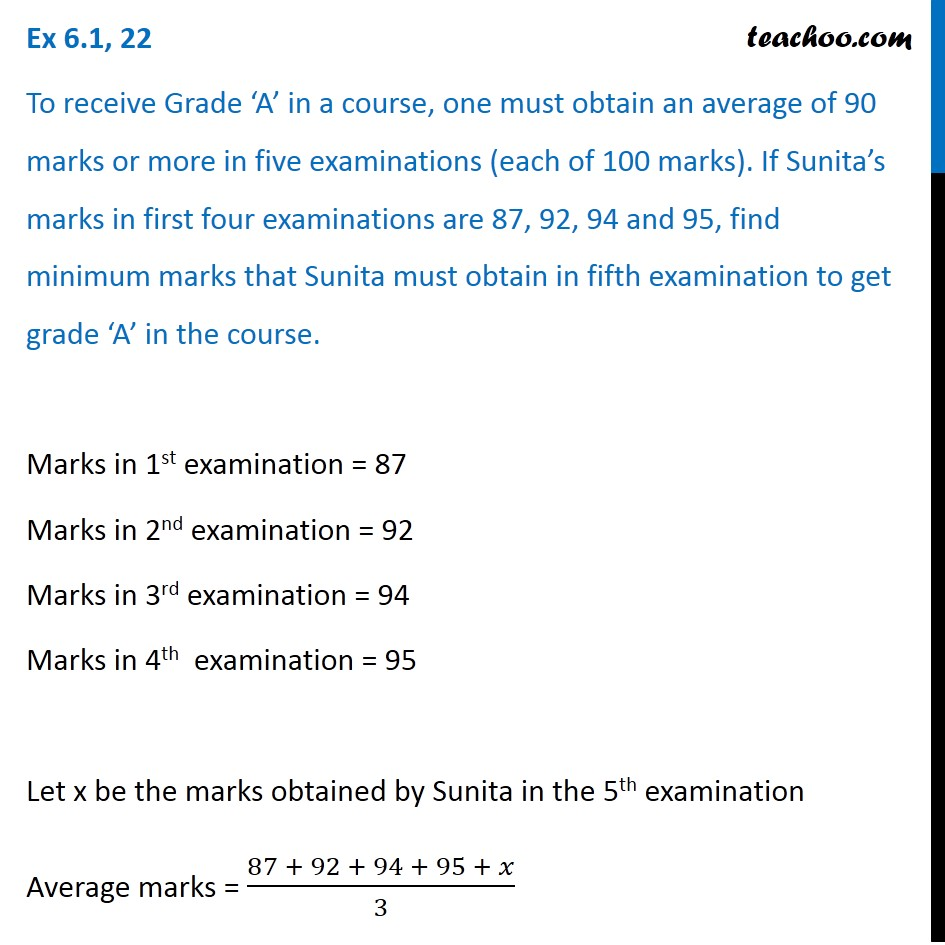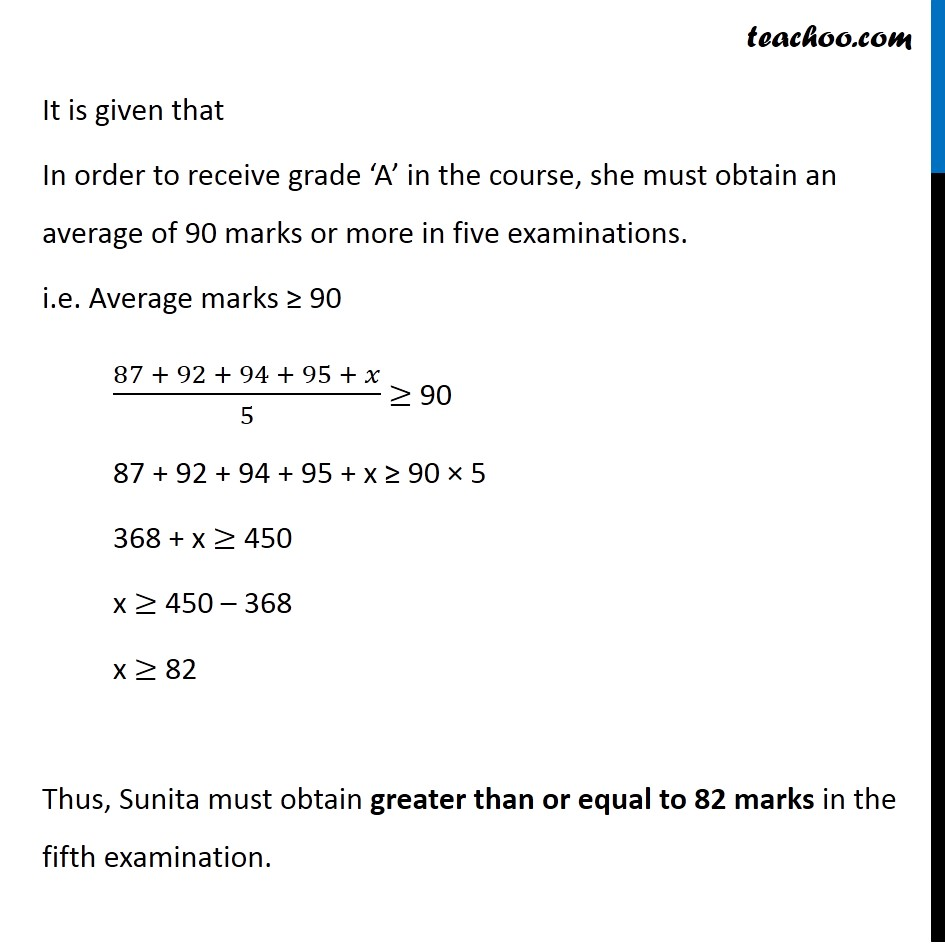Ex 6.1

Chapter 6 Class 11 Linear Inequalities (Term 2)
Serial order wise### Transcript

Ex 6.1, 22 To receive Grade ‘A’ in a course, one must obtain an average of 90 marks or more in five examinations (each of 100 marks). If Sunita’s marks in first four examinations are 87, 92, 94 and 95, find minimum marks that Sunita must obtain in fifth examination to get grade ‘A’ in the course. Marks in 1st examination = 87 Marks in 2nd examination = 92 Marks in 3rd examination = 94 Marks in 4th examination = 95 Let x be the marks obtained by Sunita in the 5th examination Average marks = (87 + 92 + 94 + 95 + 𝑥)/3 It is given that In order to receive grade ‘A’ in the course, she must obtain an average of 90 marks or more in five examinations. i.e. Average marks ≥ 90 (87 + 92 + 94 + 95 + 𝑥)/5 ≥ 90 87 + 92 + 94 + 95 + x ≥ 90 × 5 368 + x ≥ 450 x ≥ 450 – 368 x ≥ 82 Thus, Sunita must obtain greater than or equal to 82 marks in the fifth examination.